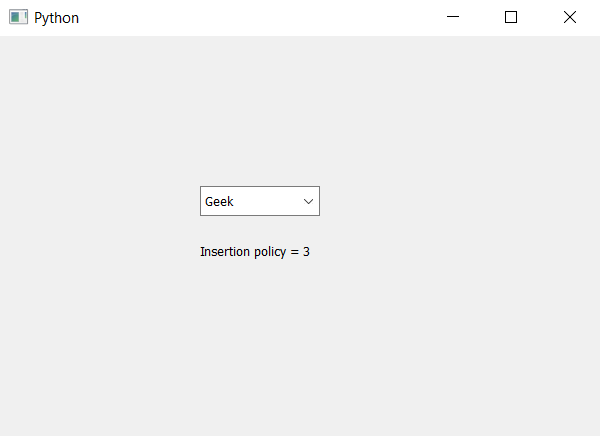Related Articles
PyQt5 – How know the insertion policy of the ComboBox
• Last Updated : 22 Apr, 2020

In this article we will see how we can know the insertion policy of the combo box. By default it insert at the bottom although we can change it any time. Insertion policy tells where will the new item when inserted by the user will get stored.

In order know the insertion policy we use `insertPolicy` method.

Syntax : combo_box.insertPolicy()

Argument : It takes no argument

Return : It returns InsertPolicy object (prints 3 for insert at bottom)

Below is the implementation

 `# importing libraries``from` `PyQt5.QtWidgets ``import` `*` `from` `PyQt5 ``import` `QtCore, QtGui``from` `PyQt5.QtGui ``import` `*` `from` `PyQt5.QtCore ``import` `*` `import` `sys`` ` ` ` `class` `Window(QMainWindow):`` ` `    ``def` `__init__(``self``):``        ``super``().__init__()`` ` `        ``# setting title``        ``self``.setWindowTitle(``"Python "``)`` ` `        ``# setting geometry``        ``self``.setGeometry(``100``, ``100``, ``600``, ``400``)`` ` `        ``# calling method``        ``self``.UiComponents()`` ` `        ``# showing all the widgets``        ``self``.show()`` ` `    ``# method for widgets``    ``def` `UiComponents(``self``):`` ` `        ``# creating a combo box widget``        ``self``.combo_box ``=` `QComboBox(``self``)`` ` `        ``# setting geometry of combo box``        ``self``.combo_box.setGeometry(``200``, ``150``, ``120``, ``30``)`` ` `        ``# geek list``        ``geek_list ``=` `[``"Geek"``, ``"Geeky Geek"``, ``"Legend Geek"``, ``"Ultra Legend Geek"``]`` ` `        ``# adding list of items to combo box``        ``self``.combo_box.addItems(geek_list)`` ` `        ``# creating editable combo box``        ``self``.combo_box.setEditable(``True``)`` ` `        ``# getting current insertion policy``        ``policy ``=` `self``.combo_box.insertPolicy()`` ` `        ``# creating label to  print the policy``        ``label ``=` `QLabel(``"Insertion policy = "` `+` `str``(policy), ``self``)`` ` `        ``# setting geometry of the label``        ``label.setGeometry(``200``, ``200``, ``200``, ``30``)`` ` ` ` `# create pyqt5 app``App ``=` `QApplication(sys.argv)`` ` `# create the instance of our Window``window ``=` `Window()`` ` `# start the app``sys.exit(App.``exec``())`

Output :Attention geek! Strengthen your foundations with the Python Programming Foundation Course and learn the basics.

To begin with, your interview preparations Enhance your Data Structures concepts with the Python DS Course. And to begin with your Machine Learning Journey, join the Machine Learning – Basic Level Course

My Personal Notes arrow_drop_up